# High School Math : Polynomial Functions

## Example Questions

### Example Question #33 : Functions And Graphs

It took Jack 25 minutes to travel 14 miles, what was Jack's average speed in mph?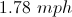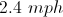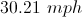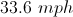Explanation: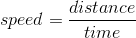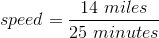* We have to change the time from minutes to hours, there are 60 minutes in one hour.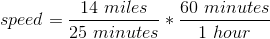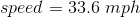### Example Question #34 : Functions And Graphs

Let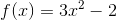and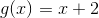.  Evaluate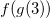.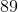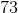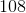Explanation:

Substituteinto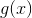, and then substitute the answer into.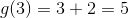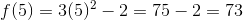### Example Question #35 : Functions And Graphs

Solve the following system of equations: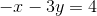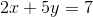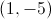Infinite solutions.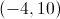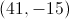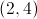Explanation:

We will solve this system of equations by Elimination.  Multiply both sides of the first equation by 2, to get: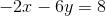Then add this new equation, to the second original equation, to get: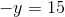or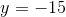Plugging this value ofback into the first original equation, gives: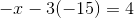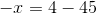or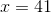### Example Question #36 : Functions And Graphs

List the transformations that have been enacted upon the following equation: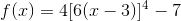vertical stretch by a factor of 4

horizontal stretch by a factor of 6

vertical translation 7 units down

horizontal translation 3 units right

vertical stretch by a factor of 4

horizontal compression by a factor of 6

vertical translation 7 units down

horizontal translation 3 units right

vertical stretch by a factor of 1/4

horizontal compression by a factor of 1/6

vertical translation 7 units down

horizontal translation 3 units right

vertical compression by a factor of 4

horizontal stretch by a factor of 6

vertical translation 7 units down

horizontal translation 3 units right

vertical stretch by a factor of 4

horizontal compression by a factor of 6

vertical translation 7 units down

horizontal translation 3 units left

vertical stretch by a factor of 4

horizontal compression by a factor of 6

vertical translation 7 units down

horizontal translation 3 units right

Explanation:

Since the equation given in the question is based off of the parent function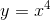, we can write the general form for transformations like this: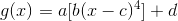determines the vertical stretch or compression factor.

• If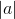is greater than 1, the function has been vertically stretched (expanded) by a factor of.
• Ifis between 0 and 1, the function has been vertically compressed by a factor of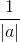.

In this case,is 4, so the function has been vertically stretched by a factor of 4.determines the horizontal stretch or compression factor.

• If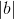is greater than 1, the function has been horizontally compressed by a factor of.
• Ifis between 0 and 1, the function has been horizontally stretched (expanded) by a factor of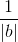.

In this case,is 6, so the function has been horizontally compressed by a factor of 6. (Remember that horizontal stretch and compression are opposite of vertical stretch and compression!)determines the horizontal translation.

• Ifis positive, the function was translatedunits right.
• Ifis negative, the function was translatedunits left.

In this case,is 3, so the function was translated 3 units right.determines the vertical translation.

• Ifis positive, the function was translatedunits up.
• Ifis negative, the function was translatedunits down.

In this case,is -7, so the function was translated 7 units down.